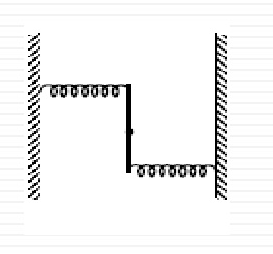# The 2 spring problemA uniform rod of mass $M$ and length $L$ is pivoted at the center. Its two ends are attached to two springs of spring constants $k$. The springs are fixed to the rigid support as shown in the figure , and the rod is free to rotate in the horizontal plane.The rod is gently pushed through small angle $\theta$ in one direction and then released. What is the frequency of oscillation?

×

Problem Loading...

Note Loading...

Set Loading...